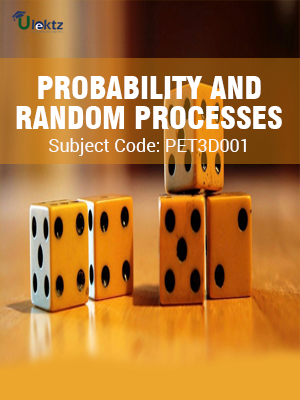•My WalletMy Order
•My Profile
•My Connections
•My Books
•My Videos
•My Tests
•My Calender
•My Messages
•My Shopping Cart
•My Orders
•Account Settings
•Help

# Book Details# PROBABILITY AND RANDOM PROCESSES

 Course Code : PET3D001 Author : uLektz University : Biju Patnaik University of Technology (BPUT) Regulation : 2016 Categories : Electronics & Communication Format :ePUB3 (DRM Protected) Type : eBook

FREE

Description :PROBABILITY AND RANDOM PROCESSES of PET3D001 covers the latest syllabus prescribed by Biju Patnaik University of Technology (BPUT) for regulation 2016. Author: uLektz, Published by uLektz Learning Solutions Private Limited.

Note : No printed book. Only ebook. Access eBook using uLektz apps for Android, iOS and Windows Desktop PC.

##### Topics
###### UNIT - I PROBABILITY

1.1 Probability: Probability introduced through Sets and Relative Frequency: Experiments and Sample Spaces, Discrete and Continuous Sample Spaces, Events

1.2 Probability Definitions and Axioms, Mathematical Model of Experiments, Probability as a Relative Frequency

1.3 Joint Probability, Conditional Probability, Total Probability, Bayes’ Theorem, Independent Events

###### UNIT - II THE RANDOM VARIABLE

2.1 The Random Variable : Definition of a Random Variable, Conditions for a Function to be a Random Variable, Discrete and Continuous, Mixed Random Variable

2.2 Distribution and Density functions, Properties, Binomial, Poisson, Uniform, Gaussian, Exponential, Rayleigh

2.3 Conditional Distribution, Methods of defining Conditioning Event, Conditional Density, Properties

###### UNIT - III OPERATION ON ONE RANDOM VARIABLE

3.1 Operation on one Random Variable: Introduction, Expected Value of a Random Variable, Function of a Random Variable

3.2 Moments about the Origin, Central Moments, Variance and Skew, Chebychev’s Inequality, Characteristic Function, Moment Generating Function

3.3 Transformations of a Random Variable: Monotonic Transformations for a Continuous Random Variable, Non-monotonic Transformations of Continuous Random Variable, Transformation of a Discrete-Random-Variable

###### UNIT - IV MULTIPLE RANDOM VARIABLES

4.1 Multiple Random Variables: Vector Random Variables, Joint Distribution Function, Properties of Joint Distribution, Marginal Distribution Functions

4.2 Conditional Distribution and Density – Point Conditioning, Conditional Distribution and Density – Interval conditioning

4.3 Statistical Independence, Sum of Two Random Variables, Sum of Several Random Variables, Central Limit Theorem, (Proof not expected). Unequal Distribution, Equal-Distributions

###### UNIT - V OPERATIONS ON MULTIPLE RANDOM VARIABLES

5.1 Operations on Multiple Random Variables: Expected Value of a Function of Random Variables: Joint Moments about the Origin, Joint Central Moments, Joint Characteristic Functions

5.2 Jointly Gaussian Random Variables: Two Random Variables case, N Random Variable case, Properties

5.3 Transformations of Multiple Random Variables, Linear Transformations of Gaussian Random Variables